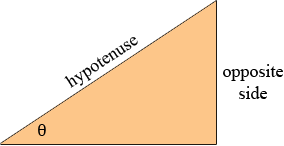SEARCH HOMEMath Central Quandaries & Querieskiran: In triangle , Sinθ = ?Hi Kiran,

In any right angle triangle if θ is the measure of one of the angles (not the right angle) then the sine of θ is the ratio of the length of the side opposite the angle θ divided by the length of the hypotenuse.PennyMath Central is supported by the University of Regina and The Pacific Institute for the Mathematical Sciences.